# Introduction of the theory of combinatorial games and a variant of the game of Nim.

In a combinatorial game there are two kinds of positions. One kind is a P-position, a previous-player-winning position. The other is an N-position, a Next-player-winning position.

Let me explain about these positions. In the followings we use the word option to mean "choice of move". Our aim is to find all the P-positions.
By the definition of P-position and N-position it is clear that they have the following properties.

Graph 1.1.Here we are going to present a bitter chocolate problem that is a variant of the game of Nim.

Definition 1.1.   Given a pieces of chocolate, where the light gray parts are sweet and the dark gray part is very bitter.Two players in turn break the chocolate (in a straight line along the grooves) and eats the piece he breaks off.The player to leave his opponent with the single bitter part is the winner.

We are going to study this problem by using examples.

Example 1.1.   We are going to use the chocolate in Graph 1.2. This has been proposed in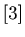.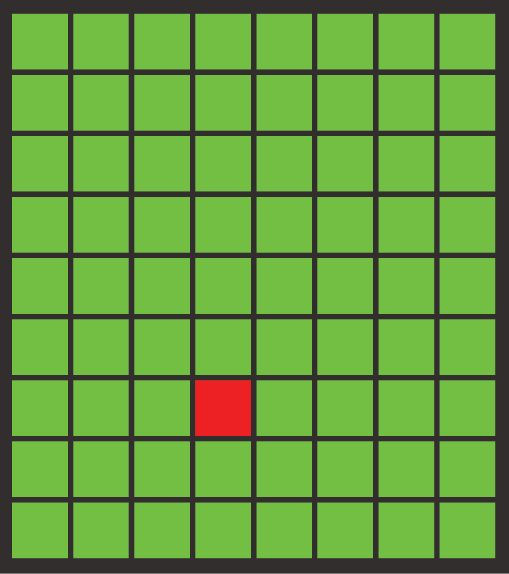Graph 1.2.                                            Graph 1.3.

It is easy to see that this problem is equivalent to the chocolate problem in Graph 1.3, and hence it is mathematically same as the game of Nim with 4 piles.
The chocolate of Graph 1.2 has 8 columns and 9 rows, but we can study the bitter chocolate problems with arbitrary numbers of rows and columns.
We can represent the position of the game with 4-numbers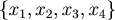, where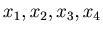are
non-negative integers. For example the position of the chocolate of Graph 1.2 is
{6,4,2,3}. When it is your turn, you choose one of the 4 coordinates and subtract a natural number that is smaller than or equal to the coordinate. These 4 coordinates are independent, i.e., you can subtract a natural number from one coordinate without affecting other coordinates.

Definition 1.2.   Here we are going to define the nim-sum. The nim-sum is the sum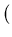in binary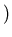neglecting all carries from one digit to another. The nim-sum of non-negative integers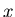and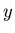is denoted by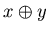.

Note that you can find an explanation of nim-sum online, if you cannot understand the definition.

Example 1.2.   We are going to calculate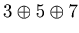. Since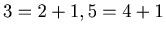and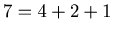,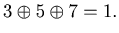Similarly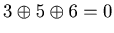, since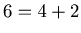.
From these calculation it is easy to see that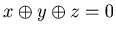implies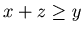,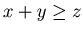and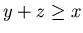.

Theorem 1.1.   In the game of Example 1.1is a P-position if and only if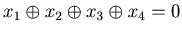.

We omit the proof, because this is a well known fact about the game of Nim. This theorem was proved in .

By Theorem 1.1 the P-positions of the chocolate problem of Example 1.1 can be obtained by mathematical theory, but in many other combinatorial games it is often the case that it is difficult to get all the P-positions mathematically, and we can get the list of P-positions only by the calculation of computers.
When we calculate P-positions by computers, one of the most important tools for that is the Grundy Number.

Here we are going to define Grundy Number using the the game of Example 1.1 as an example.
First we define a very important function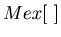.

Definition 1.3.   The Mex of a set of nonnegative integers is the least nonnegative integer not in the set.

Example 1.3.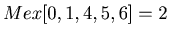and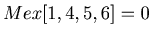.

Definition 1.4.   For any position x we denote by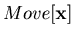the set of all the positions that players can reach directly from the position x.

Example 1.4.   We are going to use the chocolates of Example 1.1 to explain about. Let x = {1, 1, 1, 2}. Then this position is the following chocolate. See Graph 1.4.Graph 1.4.

From this position the player can reach the positions in Graph 1.5.

Graph 1.5.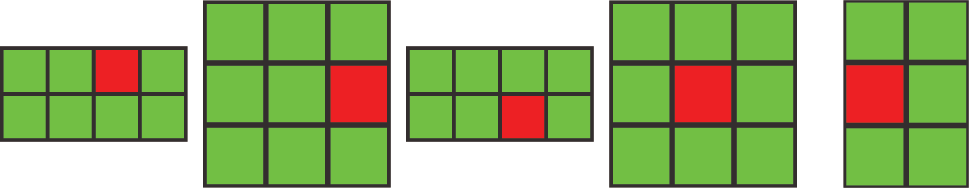Therefore= {{0, 1, 1, 2}, {1, 0, 1, 2}, {1, 1, 0, 2}, {1, 1, 1, 1}, {1, 1, 1, 0}}.

We are going to define the Grundy Number.

Definition 1.5.   Let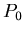be the set of positions from which the players can have no legal option.
For any position
x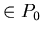we define G(x) = 0.
Let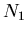be the set of positions from which the players can choose a proper option that leads to.
For any position
x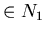we define G(x) = 1.
For any position
x we define G(x) recursively.
G(
x) =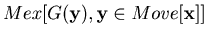.
For the details of the Grundy Number see .

By the theory of Grundy Number we know that x is a P-position if and only if G(x) = 0. Therefore we can find P-positions by calculating Grundy Number G(x)